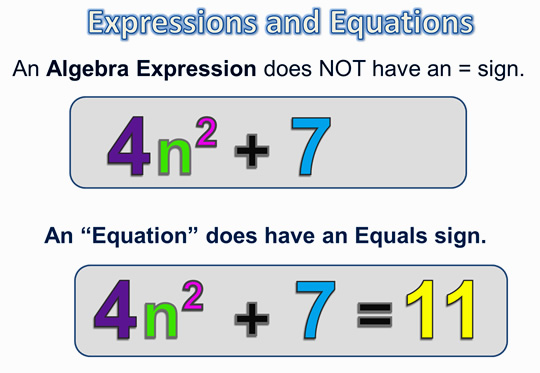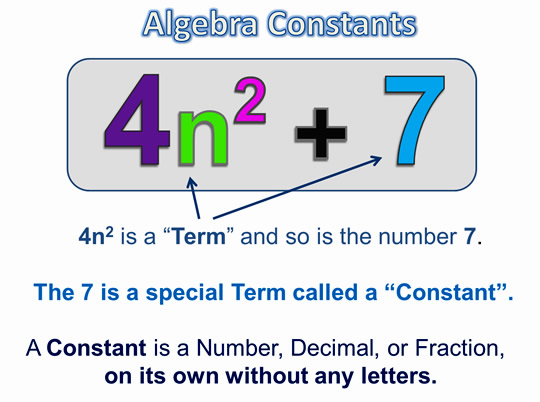# Solving algebraic equations

Welcome to JSS2 Second Term!

In today’s class, We will be discussing Solving Algebraic Equations. We hope you enjoy the class!CONTENT

• Using directed number
• Unknown on both sides
• Equations with brackets
• Equations with fractions

Using Directed Numbers

Example

(1)   Solve 25 – 9x = 2 and 12 = 9 – 3a

25 – 9x = 2

Subtract 25 from both sides

25 – 25 – 9x = 2 – 25

-9x = -23.

Divide both sides by – 9

-9x    =   – 23

– 9          -9

X = 23/9  = 2 5/9

Check

when x = 23/9

LHS = 25 – 9x 23/9 = 25 – 23 = 2 RHS

(2)    12 = 9 – 31

12 = 9 – 31

Subtract 9 – from both sides

12 – 9 = -9 – 9 – 3a

3 = -31

Divide both sides by -3

3/-3   = -31/-3

-1 = a

:. A = -1

Check when x = -1

LHS  = 12 = 9 – 3 ( -1) = RHS

LHS = 12 = 9 + 3 + RHS

LHS = 12 = 12 = RHS

SELF EVALUATION

Solve the following equations and check the Solutions.

1. 5 – 5n = 8
2. 12 + 5a = 23
3. 20 – 5t = 5

• Unknown on both sides.

If an equation has unknown terms on both sides of the equal sign, collect the unknown terms on one side and the number terms on the other side.

Worked Examples

Solve the equation.

1. 5x – 4 = 2x + 11

Solution

5x – 4 = 2x + 11

Subtract 2x from both sides of ( 1)

5x – 2x – 4 = 2x – 2x + 11

3x – 4 = 11 ……………….  ( 2 )

Add 4 to both sides of …..(2)

3x – 4 + 4 = 11 + 4

3x = 15 …………………  ( 3)

3x/3 = 15/3

X = 5.

Check: when x = 5

LHS = 5 x 5 – 4 = 25 – 4 = 21

RHS = 2 x 5 + 11 = 10 + 11 = 21 = LHS

SELF EVALUATION

Solve the following equations

1. 18 – 5f = 2f + 4
2. 5a + 6 = 2a + 20Equations with brackets

Always remove brackets before collecting terms.

Examples.

Solve

(1)   3 ( 3x – 1) = 4 ( x + 3 )

(2)   5 (x +11) + 2 (2x – 5) = 0

Solution

3 ( 3x -1) = 4 ( x + 3)…………..(1)

Remove brackets

9x – 3 = 4x + 12 …………….. ( 2)

Subtracts 4x from both sides and add 3 to both sides.

9x – 4x – 3 + 3 = 4x – 4x + 12 + 3

5x = 15 …………………………(3)

Divide both sides by 5

X = 3

Check : When x = 3

LHS = 3 ( 3 x 3 – 1 ) = 3 ( 9-1) = 3 x 8 = 24

LHS = 4 ( 3 + 3) = 4 x 6 =24 = LHS

(2)   .5  ( x + 11) + 2 ( 2x – 5 ) = 0

Remove brackets

5x + 55 + 4x – 10 = 0

Collect like terms

9x + 45 = 0

Subtract 45 from both sides

9x = -45

Divide both sides by 9

X = -5

Check : when x = -5

LHS = 5(-5 + 11) + 2 (2x (-5) – 5 )

= 5 x 6 + 2 ( -10 -5 )

=30 + 2x ( -15) = 30 – 30 = 0 = RHS

Self Evaluation

Solve the following equations and check the Solutions

1. 2 (x + 5) = 18           2.)  5(a + 2)  = 4 ( a -1)           3.)  2(y – 2) + 3 ( y – 7 ) = 0##### Equation with fractions

Always clear fractions before collecting terms.  To clear fractions, multiply both sides of the equation by the LCM of the denomination of the fractions.

Example

Solve the equations

1.

1.

Solutions

The LCM of 5 and 3 is 15.  Multiply every term by 15.

EVALUATION

Solve the following equations.

GENERAL SELF EVALUATION

1. solve 7a + 2 = 5 ( a – 4)
2. Solve the equation
3. Solve the equation

REVISION QUESTION

Solve  the  following  equations

1. 3(x + 5) – 35 = -4(2x -6)
2. 4(2x + 5) -2 (x – 3 ) = 8(2x + 4 )

3.

New General Mathematics, UBE Edition chapter 13 pgs. 110-113.

Essential Mathematics by  AJS Oluwasanmi, chapter 19pgs 200-205

WEEKEND ASSIGNMENT

1. Solve the equation 4b +24 = 0           A. -3                B. -4                            C. -5                           D. -6
2. Solve 7c – 6 = c                       A. 1                             B. 2                              C. 3                             D. 4
3. Solve 15 = 3(x – 3)                   A. 6                             B. 7                              C. 8                             D. 4
4. Solve 0 = 7 (x – 3 )                   A. 1                             B. 2                              C. 3                             D. 4
5. Solve 3m + 8 = m                   A. -1                            B. -2                            C. -3                           D. -4

THEORY

Solve the following equations.

1. 3(2x-1) =39 – 2(y + 1)

2.

We have come to the end of this class. We do hope you enjoyed the class?

Should you have any further question, feel free to ask in the comment section below and trust us to respond as soon as possible.

In our next class, we will be talking about Word Problems On Algebraic Fraction. We are eager to meet you there.

For more class notes, videos, homework help etc download our Mobile App HERE

Join ClassNotes.ng Telegram Community HERE for exclusive content and support

Don`t copy text!